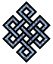#fail2ban bad ip database: ip 46.251.239.161

### | ip database | live view | stats | report | help | api key:

 ip: 46.251.239.161 hostname: 46.251.239.161 country:[DE] Germany first reported: 18.11.2018 20:56.37 GMT+0200 last reported: 20.02.2019 01:29.28 GMT+0200 time period: 93d 04h 32m 51s total reports: 10 reported by: 2 host(s) filter(s): ssh (4) ssh (6) tor exit node no badips.com db Lookup## port scan of '46.251.239.161':

[-hide]
```# Nmap 6.40 scan initiated Sun Nov 18 20:57:02 2018 as: /usr/bin/nmap -sU -sS -O 46.251.239.161
Nmap scan report for 46.251.239.161
Host is up (0.015s latency).
Not shown: 999 open|filtered ports, 993 closed ports
PORT    STATE    SERVICE
22/tcp  open     ssh
25/tcp  open     smtp
53/tcp  filtered domain
80/tcp  open     http
139/tcp filtered netbios-ssn
443/tcp open     https
445/tcp filtered microsoft-ds
123/udp open     ntp
No exact OS matches for host (If you know what OS is running on it, see http://nmap.org/submit/ ).
TCP/IP fingerprint:
OS:SCAN(V=6.40%E=4%D=11/18%OT=22%CT=1%CU=%PV=N%G=Y%TM=5BF1C48E%P=x86_64-pc-
OS:linux-gnu)SEQ(II=I)ECN(R=Y%DF=Y%TG=40%W=7210%O=M5B4NNSNW7%CC=Y%Q=)T1(R=N
OS:)T2(R=N)T3(R=N)T4(R=Y%DF=Y%TG=40%W=0%S=A%A=Z%F=R%O=%RD=0%Q=)T5(R=N)T6(R=
OS:Y%DF=Y%TG=40%W=0%S=A%A=Z%F=R%O=%RD=0%Q=)T7(R=N)U1(R=N)IE(R=Y%DFI=N%TG=40
OS:%CD=S)

OS detection performed. Please report any incorrect results at http://nmap.org/submit/ .
# Nmap done at Sun Nov 18 20:59:10 2018 -- 1 IP address (1 host up) scanned in 128.44 seconds
```
```Σ = 65 | Δt = 0.010721921920776s
```